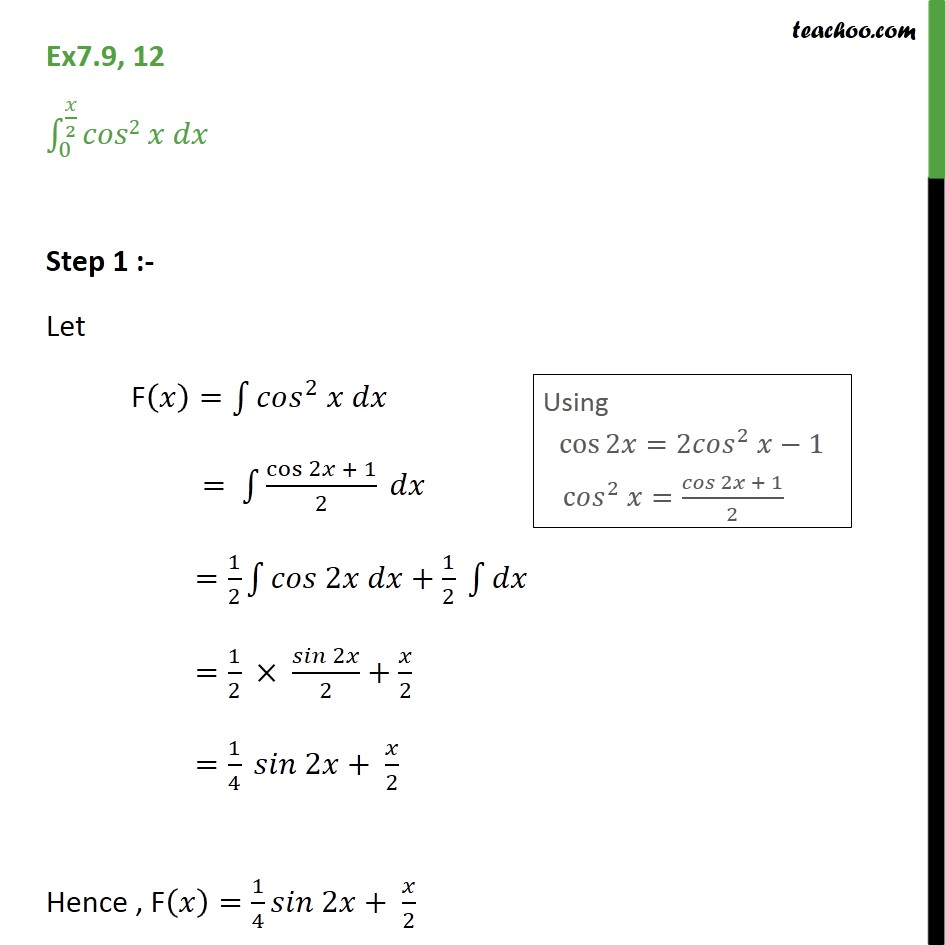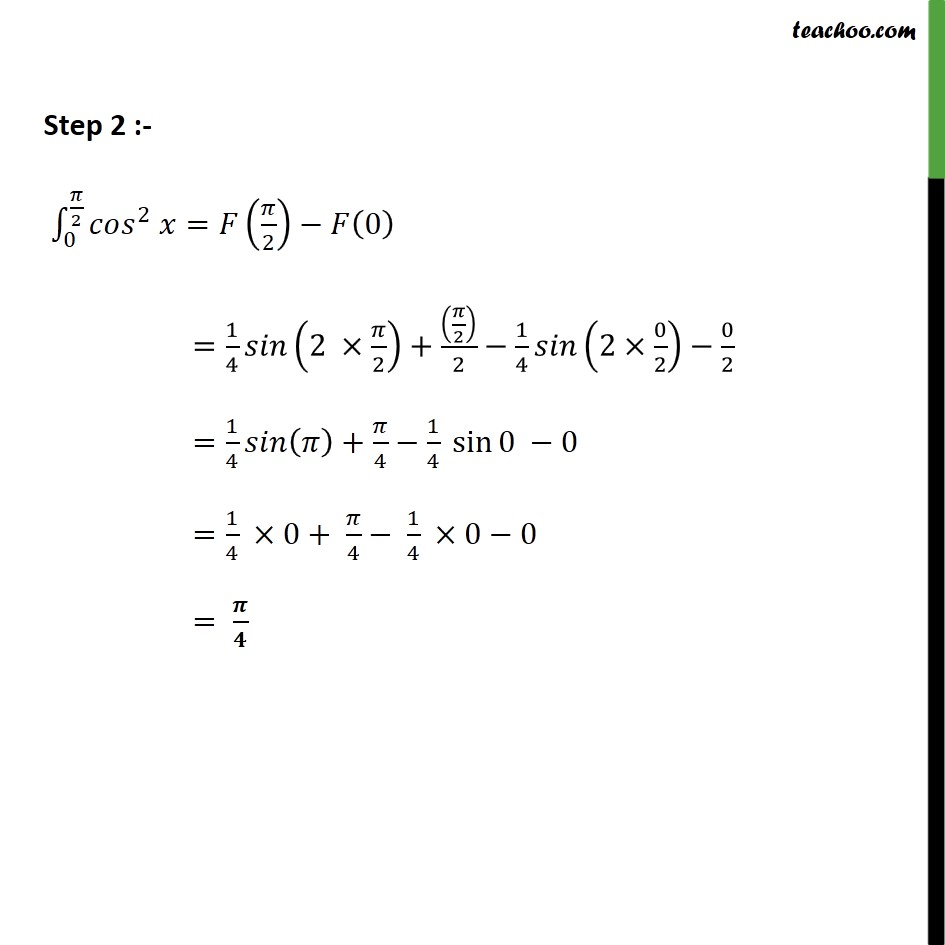Ex 7.9

Chapter 7 Class 12 Integrals
Serial order wiseIntroducing your new favourite teacher - Teachoo Black, at only ₹83 per month

### Transcript

Ex7.9, 12 0 2 2 Step 1 :- Let F = 2 = cos 2 + 1 2 = 1 2 2 + 1 2 = 1 2 2 2 + 2 = 1 4 2 + 2 Hence , F = 1 4 2 + 2 Step 2 :- 0 2 2 = 2 0 = 1 4 2 2 + 2 2 1 4 2 0 2 0 2 = 1 4 + 4 1 4 sin 0 0 = 1 4 0+ 4 1 4 0 0 =